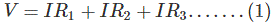# Resistors in Series and Parallel

By the end of the section, you will be able to:

• Define the term equivalent resistance
• Calculate the equivalent resistance of resistors connected in series
• Calculate the equivalent resistance of resistors connected in parallel

In Current and Resistance, we described the term ‘resistance’ and explained the basic design of a resistor. Basically, a resistor limits the flow of charge in a circuit and is an ohmic device where

Most circuits have more than one resistor. If several resistors are connected together and connected to a battery, the current supplied by the battery depends on the equivalent resistance of the circuit.

The equivalent resistance of a combination of resistors depends on both their individual values and how they are connected. The simplest combinations of resistors are series and parallel connections. In a series circuit, the output current of the first resistor flows into the input of the second resistor; therefore, the current is the same in each resistor. In a parallel circuit, all of the resistor leads on one side of the resistors are connected together and all the leads on the other side are connected together. In the case of a parallel configuration, each resistor has the same potential drop across it, and the currents through each resistor may be different, depending on the resistor. The sum of the individual currents equals the current that flows into the parallel connections.

## Resistances in Series

Suppose you have three different types of resistors – R1, R2 and R3 – and you connect them end to end as shown in the figure below, then it would be referred as resistances in series. In case of series connection, the equivalent resistance of the combination, is sum of these three electrical resistances.
That means, resistance between point A and D in the figure below, is equal to the sum of three individual resistances. The current enters in to the point A of the combination, will also leave from point D as there is no other parallel path provided in the circuit.

Now say this current is I. So this current I will pass through the resistance R1, R2 and R3. Applying Ohm’s law, it can be found that voltage drops across the resistances will be V1 = IR1, V2 = IR2 and V3 = IR3. Now, if total voltage applied across the combination of resistances in series, is V.
Then obviouslySince, sum of voltage drops across the individual resistance is nothing but the equal to applied voltage across the combination.

Now, if we consider the total combination of resistances as a single resistor of electric resistance value R, then according to Ohm’s law,
V = IR ………….(2)

Now, comparing equation (1) and (2), we get

So, the above proof shows that equivalent resistance of a combination of resistances in series is equal to the sum of individual resistance. If there were n number of resistances instead of three resistances, the equivalent resistance will be

## Resistances in Parallel

Say we have three resistors of resistance value R1, R2 and R3. These resistors are connected in such a manner that the right and left side terminal of each resistor is connected together, as shown in the figure below.

This combination is called resistances in parallel. If electric potential difference is applied across this combination, then it will draw a current I (say).
As this current will get three parallel paths through these three electrical resistances, the current will be divided into three parts. Say currents I1, I1 and I1 pass through resistor R1, R2 and R3 respectively.
Where total source current

Now, as from the figure it is clear that, each of the resistances in parallel, is connected across the same voltage source, the voltage drops across each resistor is the same, and it is same as supply voltage V (say).
Hence, according to Ohm’s law,

Now, if we consider the equivalent resistance of the combination is R.
Then,

Now putting the values of I, I1, I2 and I3 in equation (1) we get,

The above expression represents equivalent resistance of resistor in parallel. If there were n number of resistances connected in parallel, instead of three resistances, the expression of equivalent resistance would be